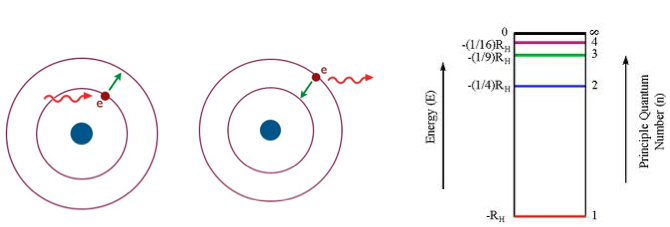# Problem: Which of the following transitions represent  absorption of a photon with the  highest frequency? n = 3 to n = 1n = 2 to n = 4n = 1 to n = 2n = 6 to n = 3n = 1 to n = 3

###### FREE Expert Solution
97% (234 ratings)
###### FREE Expert Solution

We’re being asked to determine which of the given transitions represent the absorption of a photon with the highest frequency.

In absorptionan electron gains energy and becomes excited. In this excited state, the electron moves to a higher energy level

lower energy level → higher energy level

In emission, the electron releases its excess energy to go down to a lower energy level.

higher energy level → lower energy level

The diagram below shows how much energy is required when an electron goes from 1 shell to another.Distance = Energy

97% (234 ratings)###### Problem Details

Which of the following transitions represent  absorption of a photon with the  highest frequency?

1. n = 3 to n = 1
2. n = 2 to n = 4
3. n = 1 to n = 2
4. n = 6 to n = 3
5. n = 1 to n = 3What scientific concept do you need to know in order to solve this problem?

Our tutors have indicated that to solve this problem you will need to apply the Bohr Model concept. You can view video lessons to learn Bohr Model. Or if you need more Bohr Model practice, you can also practice Bohr Model practice problems.

What is the difficulty of this problem?

Our tutors rated the difficulty ofWhich of the following transitions represent  absorption of ...as medium difficulty.

How long does this problem take to solve?

Our expert Chemistry tutor, Jules took 5 minutes and 12 seconds to solve this problem. You can follow their steps in the video explanation above.

What professor is this problem relevant for?

Based on our data, we think this problem is relevant for Professor Nagarajan's class at BSU.